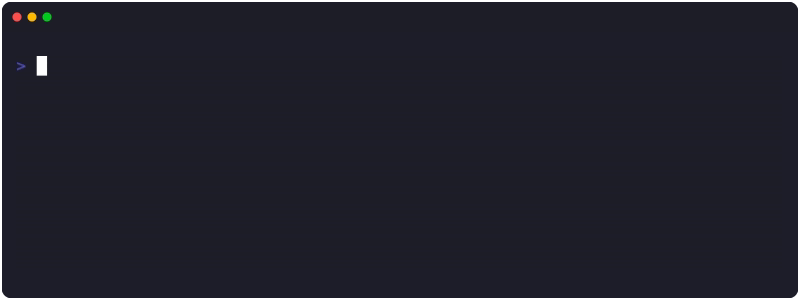# Find the Min or Max Value in a Slice (New to Go 1.21)

The latest Go release, 1.21, has introduced two new built-in functions: `min()` and `max()`. These functions have long been used in many other languages, such as Python and JavaScript, and are now welcome additions to Go.

The min() and max() functions have been introduced to simplify the process of finding the smallest and largest numbers in a set, respectively. There was plenty of talk as to whether these should be included as “built-ins” or introduced into the cmp package.

## How to use min() and max()

Note: This will only work on fixed data sets (not slices)

This method uses built-ins, see below if you’re working with slices.

 `````` 1 2 3 4 5 6 7 8 9 10 11 12 13 14 15 16 17 18 19 20 `````` ``````package main import "fmt" func main() { // Max num := max(1, 2, 3) fmt.Println("Max =", num) // Min minNum := min(1, 2, 3) fmt.Println("Min =", minNum) // Won't run: // invalid argument: myList (variable of type []int) cannot be ordered // myList := []int{1, 2, 3} // fmt.Println("Max in Slice =", max(myList)) } ``````

## Working with Slices?

The `slices` [docs] package also contains a way of finding the min and max values within our data set, as seen below. We have a simple slice with the numbers 1 to 3 and then finding both the min and max on that.

 `````` 1 2 3 4 5 6 7 8 9 10 11 12 13 14 15 16 17 18 19 `````` ``````package main import ( "fmt" "slices" ) func main() { myList := []int{1, 2, 3} // Max in Slice max := slices.Max(myList) fmt.Println("Max in Slice =", max) // Min in Slice min := slices.Min(myList) fmt.Println("Min in Slice =", min) } ``````

##Example In Action